# GcsSloop

Just do IT later.

PS：不要问我为什么不讲 PathEffect，因为这个方法在后面的Paint系列中。## Path & PathMeasure

### 构造方法

PathMeasure() 创建一个空的PathMeasure
PathMeasure(Path path, boolean forceClosed) 创建 PathMeasure 并关联一个指定的Path(Path需要已经创建完成)。

### 公共方法

void setPath(Path path, boolean forceClosed) 关联一个Path
boolean isClosed() 是否闭合
float getLength() 获取Path的长度
boolean nextContour() 跳转到下一个轮廓
boolean getSegment(float startD, float stopD, Path dst, boolean startWithMoveTo) 截取片段
boolean getPosTan(float distance, float[] pos, float[] tan) 获取指定长度的位置坐标及该点切线值
boolean getMatrix(float distance, Matrix matrix, int flags) 获取指定长度的位置坐标及该点Matrix

PathMeasure的方法也不多，接下来我们就逐一的讲解一下。

### 1.构造函数

``````  PathMeasure ()
``````

``````  PathMeasure (Path path, boolean forceClosed)
``````

1. 不论 forceClosed 设置为何种状态(true 或者 false)， 都不会影响原有Path的状态，即 Path 与 PathMeasure 关联之后，之前的的 Path 不会有任何改变。
1. forceClosed 的设置状态可能会影响测量结果，如果 Path 未闭合但在与 PathMeasure 关联的时候设置 forceClosed 为 true 时，测量结果可能会比 Path 实际长度稍长一点，获取到到是该 Path 闭合时的状态。

``````canvas.translate(mViewWidth/2,mViewHeight/2);

Path path = new Path();

path.lineTo(0,200);
path.lineTo(200,200);
path.lineTo(200,0);

PathMeasure measure1 = new PathMeasure(path,false);
PathMeasure measure2 = new PathMeasure(path,true);

Log.e("TAG", "forceClosed=false---->"+measure1.getLength());
Log.e("TAG", "forceClosed=true----->"+measure2.getLength());

canvas.drawPath(path,mDeafultPaint);
``````

log如下:

``````com.gcssloop.canvas E/TAG: forceClosed=false---->600.0
com.gcssloop.canvas E/TAG: forceClosed=true----->800.0
``````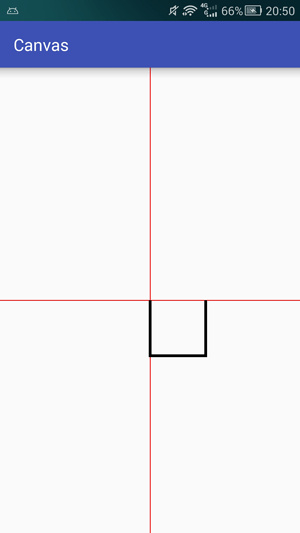• 1.我们将 Path 与两个的 PathMeasure 进行关联，并给 forceClosed 设置了不同的状态，之后绘制再绘制出来的 Path 没有任何变化，所以与 Path 与 PathMeasure进行关联并不会影响 Path 状态。
• 2.我们可以看到，设置 forceClosed 为 true 的方法比设置为 false 的方法测量出来的长度要长一点，这是由于 Path 没有闭合的缘故，多出来的距离正是 Path 最后一个点与最开始一个点之间点距离。forceClosed 为 false 测量的是当前 Path 状态的长度， forceClosed 为 true，则不论Path是否闭合测量的都是 Path 的闭合长度。

### 2.setPath、 isClosed 和 getLength

setPath 是 PathMeasure 与 Path 关联的重要方法，效果和 构造函数 中两个参数的作用是一样的。

isClosed 用于判断 Path 是否闭合，但是如果你在关联 Path 的时候设置 forceClosed 为 true 的话，这个方法的返回值则一定为true。

getLength 用于获取 Path 的总长度，在之前的测试中已经用过了。

### 3.getSegment

getSegment 用于获取Path的一个片段，方法如下：

``````boolean getSegment (float startD, float stopD, Path dst, boolean startWithMoveTo)
``````

startD 开始截取位置距离 Path 起点的长度 取值范围: 0 <= startD < stopD <= Path总长度
stopD 结束截取位置距离 Path 起点的长度 取值范围: 0 <= startD < stopD <= Path总长度
dst 截取的 Path 将会添加到 dst 中 注意: 是添加，而不是替换
startWithMoveTo 起始点是否使用 moveTo 用于保证截取的 Path 第一个点位置不变
• 如果 startD、stopD 的数值不在取值范围 [0, getLength] 内，或者 startD == stopD 则返回值为 false，不会改变 dst 内容。
• 如果在安卓4.4或者之前的版本，在默认开启硬件加速的情况下，更改 dst 的内容后可能绘制会出现问题，请关闭硬件加速或者给 dst 添加一个单个操作，例如: dst.rLineTo(0, 0)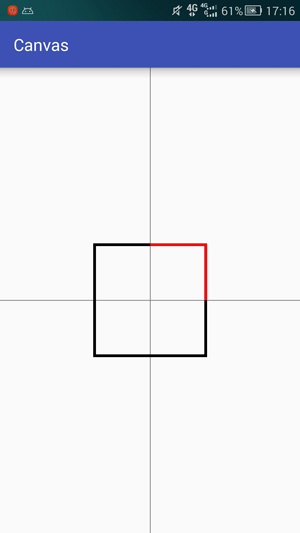``````canvas.translate(mViewWidth / 2, mViewHeight / 2);          // 平移坐标系

Path path = new Path();                                     // 创建Path并添加了一个矩形

Path dst = new Path();                                      // 创建用于存储截取后内容的 Path

PathMeasure measure = new PathMeasure(path, false);         // 将 Path 与 PathMeasure 关联

// 截取一部分存入dst中，并使用 moveTo 保持截取得到的 Path 第一个点的位置不变
measure.getSegment(200, 600, dst, true);

canvas.drawPath(dst, mDeafultPaint);                        // 绘制 dst
``````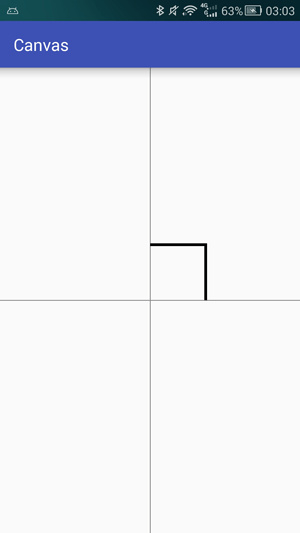``````canvas.translate(mViewWidth / 2, mViewHeight / 2);          // 平移坐标系

Path path = new Path();                                     // 创建Path并添加了一个矩形

Path dst = new Path();                                      // 创建用于存储截取后内容的 Path
dst.lineTo(-300, -300);                                     // <--- 在 dst 中添加一条线段

PathMeasure measure = new PathMeasure(path, false);         // 将 Path 与 PathMeasure 关联

measure.getSegment(200, 600, dst, true);                   // 截取一部分 并使用 moveTo 保持截取得到的 Path 第一个点的位置不变

canvas.drawPath(dst, mDeafultPaint);                        // 绘制 Path
``````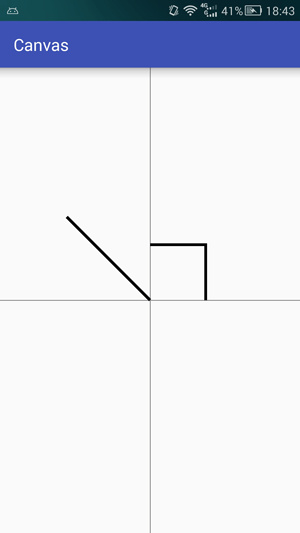``````canvas.translate(mViewWidth / 2, mViewHeight / 2);          // 平移坐标系

Path path = new Path();                                     // 创建Path并添加了一个矩形

Path dst = new Path();                                      // 创建用于存储截取后内容的 Path
dst.lineTo(-300, -300);                                     // 在 dst 中添加一条线段

PathMeasure measure = new PathMeasure(path, false);         // 将 Path 与 PathMeasure 关联

measure.getSegment(200, 600, dst, false);                   // <--- 截取一部分 不使用 startMoveTo, 保持 dst 的连续性

canvas.drawPath(dst, mDeafultPaint);                        // 绘制 Path
``````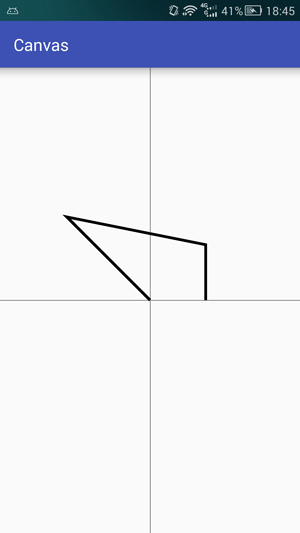true 保证截取得到的 Path 片段不会发生形变
false 保证存储截取片段的 Path(dst) 的连续性

### 4.nextContour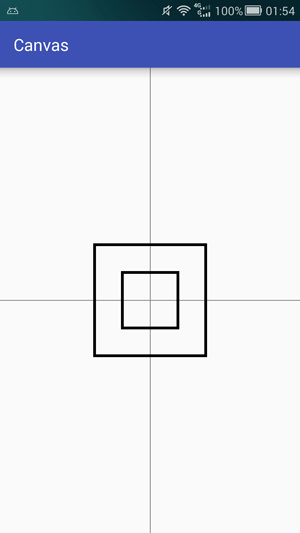``````canvas.translate(mViewWidth / 2, mViewHeight / 2);      // 平移坐标系

Path path = new Path();

path.addRect(-100, -100, 100, 100, Path.Direction.CW);  // 添加小矩形
path.addRect(-200, -200, 200, 200, Path.Direction.CW);  // 添加大矩形

canvas.drawPath(path,mDeafultPaint);                    // 绘制 Path

PathMeasure measure = new PathMeasure(path, false);     // 将Path与PathMeasure关联

float len1 = measure.getLength();                       // 获得第一条路径的长度

measure.nextContour();                                  // 跳转到下一条路径

float len2 = measure.getLength();                       // 获得第二条路径的长度

Log.i("LEN","len1="+len1);                              // 输出两条路径的长度
Log.i("LEN","len2="+len2);
``````

log输出结果:

``````com.gcssloop.canvas I/LEN: len1=800.0
com.gcssloop.canvas I/LEN: len2=1600.0
``````

• 1.曲线的顺序与 Path 中添加的顺序有关。
• 2.getLength 获取到到是当前一条曲线分长度，而不是整个 Path 的长度。
• 3.getLength 等方法是针对当前的曲线(其它方法请自行验证)。

#### 5.getPosTan

``````boolean getPosTan (float distance, float[] pos, float[] tan)
``````

false 表示失败，pos 和 tan 不会改变
distance 距离 Path 起点的长度 取值范围: 0 <= distance <= getLength
pos 该点的坐标值 当前点在画布上的位置，有两个数值，分别为x，y坐标。
tan 该点的正切值 当前点在曲线上的方向，使用 Math.atan2(tan, tan) 获取到正切角的弧度值。

`tan` 是用来判断 Path 上趋势的，即在这个位置上曲线的走向，请看下图示例，注意箭头的方向:``````private float currentValue = 0;     // 用于纪录当前的位置,取值范围[0,1]映射Path的整个长度

private float[] pos;                // 当前点的实际位置
private float[] tan;                // 当前点的tangent值,用于计算图片所需旋转的角度
private Bitmap mBitmap;             // 箭头图片
private Matrix mMatrix;             // 矩阵,用于对图片进行一些操作
``````

``````private void init(Context context) {
pos = new float;
tan = new float;
BitmapFactory.Options options = new BitmapFactory.Options();
options.inSampleSize = 2;       // 缩放图片
mBitmap = BitmapFactory.decodeResource(context.getResources(), R.drawable.arrow, options);
mMatrix = new Matrix();
}
``````

``````
canvas.translate(mViewWidth / 2, mViewHeight / 2);      // 平移坐标系

Path path = new Path();                                 // 创建 Path

path.addCircle(0, 0, 200, Path.Direction.CW);           // 添加一个圆形

PathMeasure measure = new PathMeasure(path, false);     // 创建 PathMeasure

currentValue += 0.005;                                  // 计算当前的位置在总长度上的比例[0,1]
if (currentValue >= 1) {
currentValue = 0;
}

measure.getPosTan(measure.getLength() * currentValue, pos, tan);        // 获取当前位置的坐标以及趋势

mMatrix.reset();                                                        // 重置Matrix
float degrees = (float) (Math.atan2(tan, tan) * 180.0 / Math.PI); // 计算图片旋转角度

mMatrix.postRotate(degrees, mBitmap.getWidth() / 2, mBitmap.getHeight() / 2);   // 旋转图片
mMatrix.postTranslate(pos - mBitmap.getWidth() / 2, pos - mBitmap.getHeight() / 2);   // 将图片绘制中心调整到与当前点重合

canvas.drawPath(path, mDeafultPaint);                                   // 绘制 Path
canvas.drawBitmap(mBitmap, mMatrix, mDeafultPaint);                     // 绘制箭头

invalidate();                                                           // 重绘页面
``````

• 1.通过 `tan` 得值计算出图片旋转的角度，tan 是 tangent 的缩写，即中学中常见的正切， 其中tan是邻边边长，tan是对边边长，而Math中 `atan2` 方法是根据正切是数值计算出该角度的大小,得到的单位是弧度(取值范围是 -pi 到 pi)，所以上面又将弧度转为了角度。
• 2.通过 `Matrix` 来设置图片对旋转角度和位移，这里使用的方法与前面讲解过对 canvas操作 有些类似，对于 `Matrix` 会在后面专一进行讲解，敬请期待。
• 3.页面刷新，页面刷新此处是在 onDraw 里面调用了 invalidate 方法来保持界面不断刷新，但并不提倡这么做，正确对做法应该是使用 线程 或者 ValueAnimator 来控制界面的刷新，关于控制页面刷新这一部分会在后续的 动画部分 详细讲解，同样敬请期待。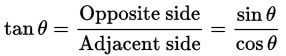tan = cos = 邻边(单位圆x坐标)
tan = sin = 对边(单位圆y坐标)

`sin／cos`理解: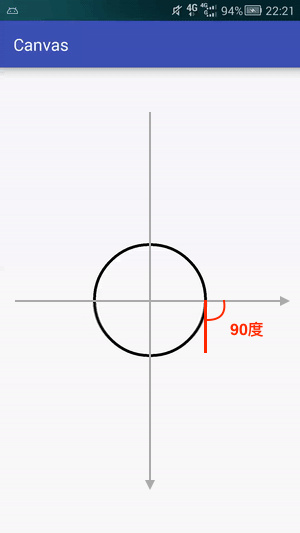sin90 = 1，cos90 = 0
tan = cos = 0
tan = sin = 1

`对边／邻边` 理解(单位圆上坐标):tan30 = 对边／邻边 = AB／OA = B点y坐标／B点x坐标

sin30 = 对边／斜边 = AB／OB = AB = B点y坐标 (单位圆边上任意一点距离圆心距离均为1，故OB = 1)
cos30 = 邻边／斜边 = OA／OB = OA = B点x坐标

sin = 该角度在单位圆上对应点的y坐标
cos = 该角度在单位圆上对应点的x坐标

tan = x
tan = y

tan = x = 0
tan = y = 1

PS: 使用 Math.atan2(tan, tan) 将 `tan` 转化为角(单位为弧度)的时候要注意参数顺序。

### 6.getMatrix

``````boolean getMatrix (float distance, Matrix matrix, int flags)
``````

distance 距离 Path 起点的长度 取值范围: 0 <= distance <= getLength
matrix 根据 falgs 封装好的matrix 会根据 flags 的设置而存入不同的内容
flags 规定哪些内容会存入到matrix中 可选择
POSITION_MATRIX_FLAG(位置)
ANGENT_MATRIX_FLAG(正切)

``````measure.getMatrix(distance, matrix, PathMeasure.TANGENT_MATRIX_FLAG | PathMeasure.POSITION_MATRIX_FLAG);
``````

``````Path path = new Path();                                 // 创建 Path

path.addCircle(0, 0, 200, Path.Direction.CW);           // 添加一个圆形

PathMeasure measure = new PathMeasure(path, false);     // 创建 PathMeasure

currentValue += 0.005;                                  // 计算当前的位置在总长度上的比例[0,1]
if (currentValue >= 1) {
currentValue = 0;
}

// 获取当前位置的坐标以及趋势的矩阵
measure.getMatrix(measure.getLength() * currentValue, mMatrix, PathMeasure.TANGENT_MATRIX_FLAG | PathMeasure.POSITION_MATRIX_FLAG);

mMatrix.preTranslate(-mBitmap.getWidth() / 2, -mBitmap.getHeight() / 2);   // <-- 将图片绘制中心调整到与当前点重合(注意:此处是前乘pre)

canvas.drawPath(path, mDeafultPaint);                                   // 绘制 Path
canvas.drawBitmap(mBitmap, mMatrix, mDeafultPaint);                     // 绘制箭头

invalidate();                                                           // 重绘页面
``````

• 1.对 `matrix` 的操作必须要在 `getMatrix` 之后进行，否则会被 `getMatrix` 重置而导致无效。
• 2.矩阵对旋转角度默认为图片的左上角，我们此处需要使用 `preTranslate` 调整为图片中心。
• 3.pre(矩阵前乘) 与 post(矩阵后乘) 的区别，此处请等待后续的文章或者自行搜索。

## Path & SVG

SVG 是一种矢量图，内部用的是 xml 格式化存储方式存储这操作和数据，你完全可以将 SVG 看作是 Path 的各项操作简化书写后的存储格式。

Path 和 SVG 结合通常能诞生出一些奇妙的东西，如下:SVG 转 Path 的解析可以用这个库 -> AndroidSVG

## Path使用技巧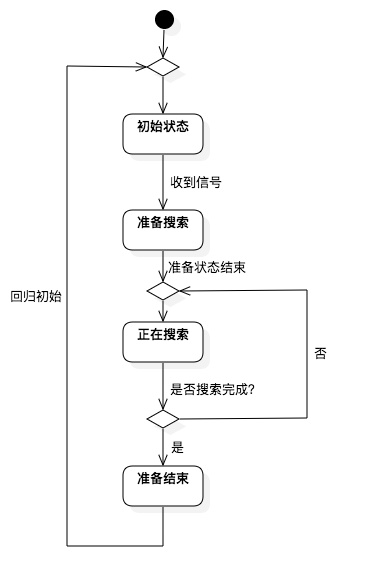### Path 划分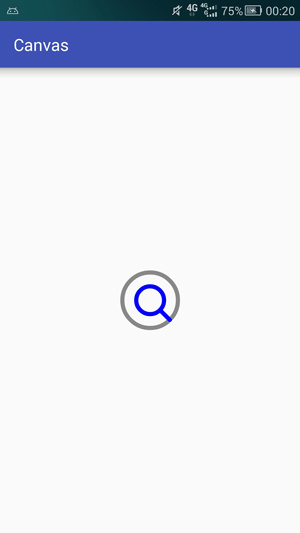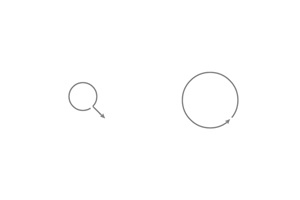### 动画状态与时间关联

PS: 本来不想提前暴露这个的，准备偷偷留到动画部分(｡-_-｡) 但实在是没有优雅的替代方案了。

### 最终效果### 源码

#### 戳这里查看源码

PS: 本代码仅作为示例使用，还有诸多不足，如 自定义属性，视图大小， 点击事件， 监听回调 等，并不适合直接使用，有需要的可以自行补足相关内容。

¥ 点击赞助

## 欢迎关注我的微信公众号CustomView

CustomView